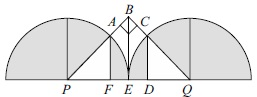# User Forum

Subject :IMO    Class : Class 8

The given figure is made up of a triangle and two identical semi-circles. P and Q are the centres of semicircles. If EP is the radius of the semicircle and measures 14 cm, then find the total shaded area.A156 cm2
B108 cm2
C250 cm2
DNone of these

The solution to this question by sof Olympiad trainer is: Area of the 2 semi-circles = 2(Â½ Ï 142) cm2 = 22/7 x 14 x 14 = 616 cm2 So, the answer is - Dbut both the semicircles are not completely shaded???????

Class : Class 8

## Ans 2:

Class : Class 10
The solution explanation is wrong.two semicircles are not shaded only.

Class : Class 8